Function Repository Resource:

# Alphametic

Solve an alphametic puzzle

Contributed by: Peter Valko
 ResourceFunction["Alphametic"][string] finds a solution to the alphametic puzzle string or returns an empty list if no such exists.

## Details and Options

ResourceFunction["Alphametic"][string,n] returns n solutions if possible.
ResourceFunction["Alphametic"][string,All] returns all solutions.
The string must contain one and only one equal sign.
Upper and lower case are treated as different letters.
The solver uses interval arithmetic and backtrack search.

## Examples

### Basic Examples (3)

Solve the classic alphametic puzzle "send+more=money":

 In:=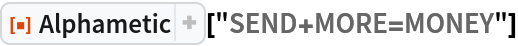Out=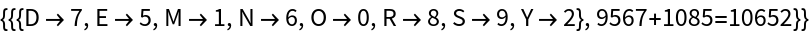This has a unique solution:

 In:=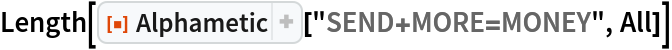Out=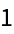In this example, there are 1200 solutions:

 In:=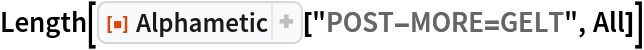Out=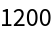If a puzzle has no solution, then an empty list is returned:

 In:=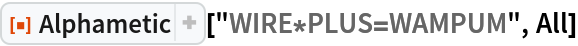Out=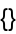In:=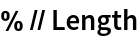Out=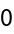### Basic Examples (1)

Parentheses and exponentiation operations are allowed:

 In:=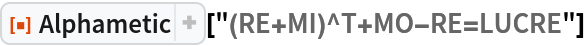Out=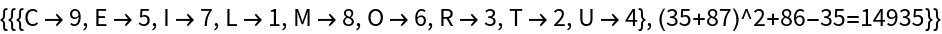Peter Valko

## Version History

• 2.0.0 – 15 April 2020
• 1.0.0 – 08 April 2020